# Boost converter works with wide-range negative-input supply

## EL7515

Mike Wong, Intersil

EDN

Assume that a design requires positive voltage, but only a negative-voltage power source is available. Using a standard boost-converter IC in the circuit of Figure 1, you can efficiently generate a positive voltage from a negative source. The boost converter generates an output voltage that’s higher than the input voltage. Because the output voltage – 5 V in this example – is higher than the negative-input-voltage ground level, the circuit does not violate the boost-converter principle.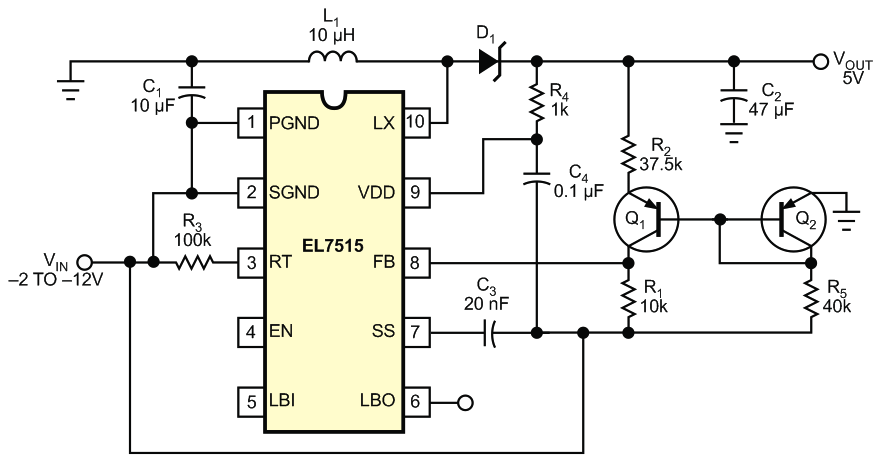Figure 1. By using its ground terminals as the negative-voltage input, a boost converter can efficiently generate a positive output voltage.

The circuit in Figure 1 uses the EL7515, a standard boost converter. The ground pins of the converter IC connect to a negative-voltage input source. Ground becomes the “positive” input source. VOUT is as follows: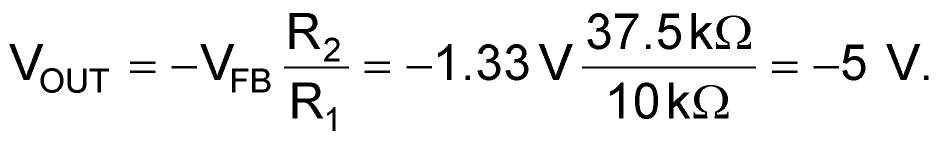The Q1 and Q2 pnp transistors form a translator that scales the 5 V output voltage (referred to ground) to a feedback voltage referred to the negative input. The transistor pair also eliminates temperature-change and voltage-drop effects. As the negative input voltage decreases, Q2 runs at an increasingly higher current than Q1, causing additional transistor-offset mismatch.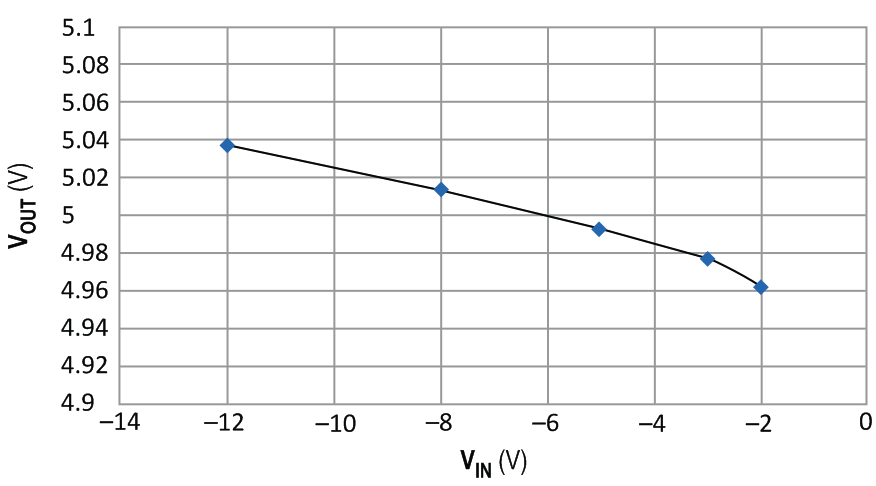Figure 2. The line regulation is within ±40 mV over the full range of negative inputs.

For optimal line regulation, you should set Q1 and Q2 to operate at the same currents with the nominal input-voltage applied. Figure 2 shows the line-regulation results. The maximum output-to-input voltage difference must be within the boost converter’s internal power FET drain-to-source breakdown voltage (VDS).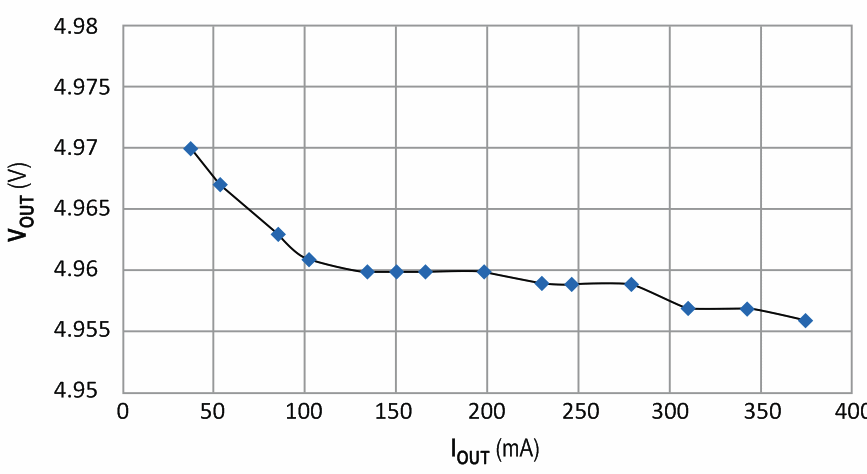Figure 3. The output voltage varies by less than 14 mV over the full range of output currents.

For the EL7515, the maximum VDS is 18 V. For the 5 V output, the minimum (most negative) input voltage is –12 V. A 1 V safety margin compensates for the D1 diode drop and any voltage spikes on the drain of the power FET. Figure 3 shows the load-regulation test results.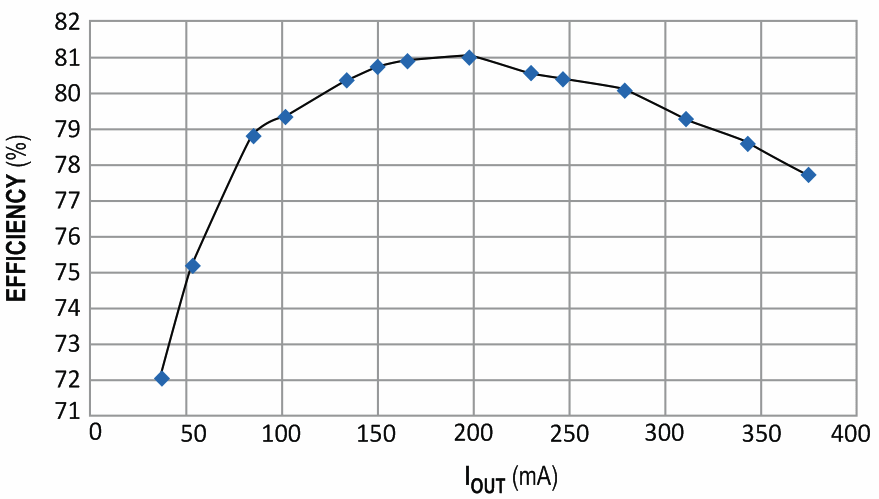Figure 4. The efficiency of the circuit peaks at 81% for medium output current (200 mA).

The maximum output current is a function of the input-to-output voltage ratio and current- limit setting of the boost converter. As Figure 4 shows, the circuit yields greater than 80% efficiency at 200-mA output.

EDN

You may have to register before you can post comments and get full access to forum.
 User Name Remember Me? Password